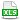HomeTechniques and Tips@RISK: General QuestionsHow Many Iterations Do I Need?

# 2.6. How Many Iterations Do I Need?

Applies to: @RISK for Excel 5.x–7.x

How many iterations do I need to run in my simulation so that the estimate of the mean is calculated within a specific confidence interval?

The answer depends on whether you're using traditional Monte Carlo sampling or the default Latin Hypercube sampling.

Monte Carlo Sampling:

The attached example, ConfIntervalWidth2.xls, uses traditional Monte Carlo sampling. Let's suppose that we want to use simulation to estimate the mean of the output in cell B11 and be accurate within 5 units 95% of the time. The number of iterations needed to meet these requirements can be calculated using the following formula:

n = [ zα/2 S / E ] ²

In this formula,

• n is the number of iterations needed.
• S is the estimated standard deviation of the output.
• E is the desired margin of error (in this case, 5 units). The width of the confidence interval is twice the margin of error.
• zα/2 is the critical value of the normal distribution for α/2, the z value such that the area of the right-hand tail is α/2. It is the number that satisfies P(Z>zα/2) = α/2, where Z follows a normal distribution with mean 0 and standard deviation 1. α/2 can be found by setting the desired confidence level equal to 100(1-α) and solving for α.

For a 95% confidence level, as shown in the attached example, 95 = 100(1-α).  Then α is 0.05 and α/2 is 0.025. To compute zα/2 in Excel, use the NORMSINV function and enter =NORMSINV(1-α/2, 0, 1). Cell E13 of the attached example shows a Z value of approximately 1.96 for a 95% confidence interval.

To obtain an estimate for the standard deviation of the output, the @RISK statistics function RiskStdDev was placed in cell B14 and a simulation was run with just 100 iterations. This gave us a standard deviation of approximately 53.5. If we plug the above information into our formula, we get

n = [ 1.96 × 53.5 / 5 ] ² = 440

Thus, if you use Monte Carlo sampling, you should run at least 440 iterations to be 95% sure that your estimate of the mean of the output in cell B11 is accurate within ±5 units.

Latin Hypercube Sampling:

The Latin Hypercube method produces sample means that are much closer together for the same number of iterations. With the Latin Hypercube method, a smaller number of iterations will be sufficient to produce means within the desired confidence interval, but there's no simple calculation to predict the necessary number. See Latin Hypercube Versus Monte Carlo Sampling, and the section "Confidence Interval with Latin Hypercube Sampling" in Confidence Intervals in @RISK.

Convergence:

Rather than try to pre-compute the necessary number of iterations, you may find it simpler just to set your convergence criteria and let @RISK run until the desired level of confidence has been reached. In Simulation Settings, on the General tab, set the number of iterations to Automatic. Then on the Convergence tab, set your convergence criteria. Notice that the margin of error ("Convergence Tolerance") is set to a percentage of the statistic being estimated, not to a number of units.

Last edited: 2015-06-08

•ConfIntervalWidth2.xls